Test: Theorems of Integral Calculus- 1

# Test: Theorems of Integral Calculus- 1

Test Description

## 20 Questions MCQ Test GATE Mechanical (ME) 2023 Mock Test Series | Test: Theorems of Integral Calculus- 1

Test: Theorems of Integral Calculus- 1 for Civil Engineering (CE) 2023 is part of GATE Mechanical (ME) 2023 Mock Test Series preparation. The Test: Theorems of Integral Calculus- 1 questions and answers have been prepared according to the Civil Engineering (CE) exam syllabus.The Test: Theorems of Integral Calculus- 1 MCQs are made for Civil Engineering (CE) 2023 Exam. Find important definitions, questions, notes, meanings, examples, exercises, MCQs and online tests for Test: Theorems of Integral Calculus- 1 below.
Solutions of Test: Theorems of Integral Calculus- 1 questions in English are available as part of our GATE Mechanical (ME) 2023 Mock Test Series for Civil Engineering (CE) & Test: Theorems of Integral Calculus- 1 solutions in Hindi for GATE Mechanical (ME) 2023 Mock Test Series course. Download more important topics, notes, lectures and mock test series for Civil Engineering (CE) Exam by signing up for free. Attempt Test: Theorems of Integral Calculus- 1 | 20 questions in 60 minutes | Mock test for Civil Engineering (CE) preparation | Free important questions MCQ to study GATE Mechanical (ME) 2023 Mock Test Series for Civil Engineering (CE) Exam | Download free PDF with solutions
 1 Crore+ students have signed up on EduRev. Have you?
Test: Theorems of Integral Calculus- 1 - Question 1

### As x is increased from – ∞ to ∞ , the function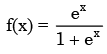Detailed Solution for Test: Theorems of Integral Calculus- 1 - Question 1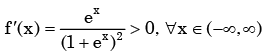Test: Theorems of Integral Calculus- 1 - Question 2

###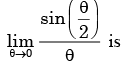Detailed Solution for Test: Theorems of Integral Calculus- 1 - Question 2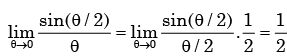Test: Theorems of Integral Calculus- 1 - Question 3

###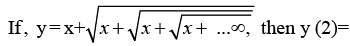Detailed Solution for Test: Theorems of Integral Calculus- 1 - Question 3

Given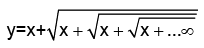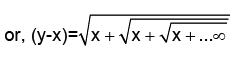Square both side, we get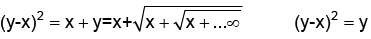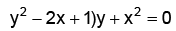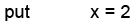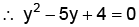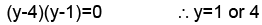Test: Theorems of Integral Calculus- 1 - Question 4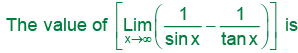Detailed Solution for Test: Theorems of Integral Calculus- 1 - Question 4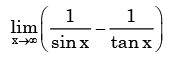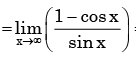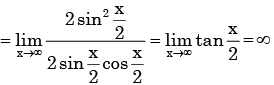Test: Theorems of Integral Calculus- 1 - Question 5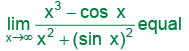Detailed Solution for Test: Theorems of Integral Calculus- 1 - Question 5

cos x and sin x are finite whatever x may be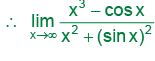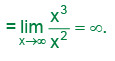Test: Theorems of Integral Calculus- 1 - Question 6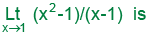Detailed Solution for Test: Theorems of Integral Calculus- 1 - Question 6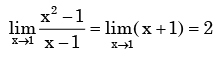Test: Theorems of Integral Calculus- 1 - Question 7

Which of the following functions is not differentiable in the domain [-1,1]?

Detailed Solution for Test: Theorems of Integral Calculus- 1 - Question 7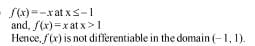Test: Theorems of Integral Calculus- 1 - Question 8

If f(x) =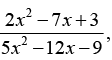then limx-►3 f(x) will be

Detailed Solution for Test: Theorems of Integral Calculus- 1 - Question 8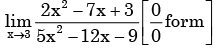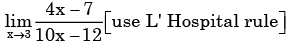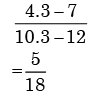Test: Theorems of Integral Calculus- 1 - Question 9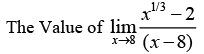Detailed Solution for Test: Theorems of Integral Calculus- 1 - Question 9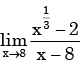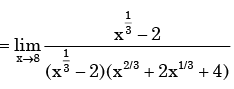= 1/12

Test: Theorems of Integral Calculus- 1 - Question 10

What should be the value of λ such that the function defined below is continuous at x = π/2?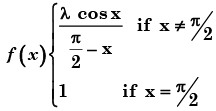Detailed Solution for Test: Theorems of Integral Calculus- 1 - Question 10

By the given condition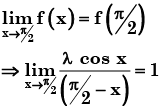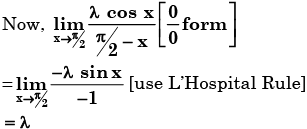From (1), λ = 1

Test: Theorems of Integral Calculus- 1 - Question 11

The value of the function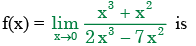Detailed Solution for Test: Theorems of Integral Calculus- 1 - Question 11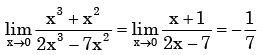Test: Theorems of Integral Calculus- 1 - Question 12

Consider the function f(x) = |x|3, where x is real. Then the function f(x) at x = 0 is

Detailed Solution for Test: Theorems of Integral Calculus- 1 - Question 12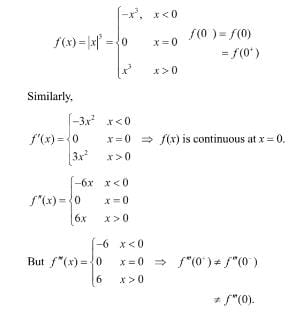Test: Theorems of Integral Calculus- 1 - Question 13

The expression e–ln x for x > 0 is equal to

Detailed Solution for Test: Theorems of Integral Calculus- 1 - Question 13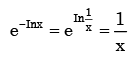Test: Theorems of Integral Calculus- 1 - Question 14

Consider the following two statements about the function f(x) = |x|

P: f(x) is continuous for all real values of x

Q: f(x) is differentiable for all real values of x

Which of the f oll owi ng is TRU E?

Detailed Solution for Test: Theorems of Integral Calculus- 1 - Question 14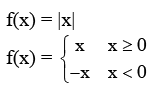The graph of f(x) is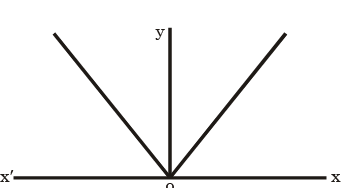f(x) is continuous for all real values of x   Lim |x| = Lim |x| = 0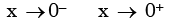as can be seen from graph of |x|.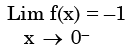and  Lim f(x) = +1 as can be seen from graph of |x|

x → 0+

Left deriva tive ≠ Rig ht derivative

So |x| is continuous but not differentiable at x = 0.

Test: Theorems of Integral Calculus- 1 - Question 15

What is the value of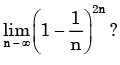Detailed Solution for Test: Theorems of Integral Calculus- 1 - Question 15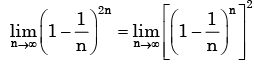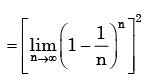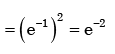Test: Theorems of Integral Calculus- 1 - Question 16

The integral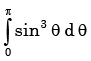is given by

Detailed Solution for Test: Theorems of Integral Calculus- 1 - Question 16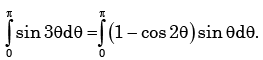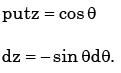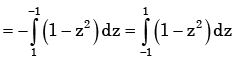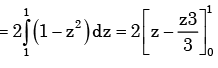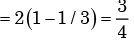Test: Theorems of Integral Calculus- 1 - Question 17

Which one of the following function is strictly bounded?

Detailed Solution for Test: Theorems of Integral Calculus- 1 - Question 17

For a strictly bounded function f(x), limit should be finite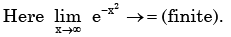Test: Theorems of Integral Calculus- 1 - Question 18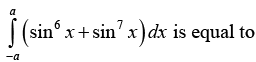Detailed Solution for Test: Theorems of Integral Calculus- 1 - Question 18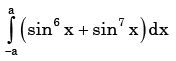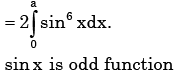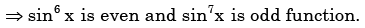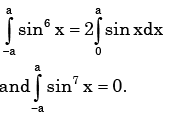Test: Theorems of Integral Calculus- 1 - Question 19

Which of the following integrals is unbounded?

Detailed Solution for Test: Theorems of Integral Calculus- 1 - Question 19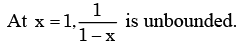Test: Theorems of Integral Calculus- 1 - Question 20

What is the value of the definite integral,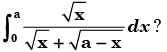Detailed Solution for Test: Theorems of Integral Calculus- 1 - Question 20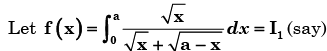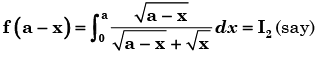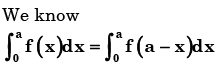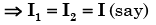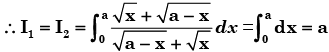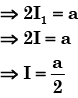## GATE Mechanical (ME) 2023 Mock Test Series

26 docs|239 tests
 Use Code STAYHOME200 and get INR 200 additional OFF Use Coupon Code
Information about Test: Theorems of Integral Calculus- 1 Page
In this test you can find the Exam questions for Test: Theorems of Integral Calculus- 1 solved & explained in the simplest way possible. Besides giving Questions and answers for Test: Theorems of Integral Calculus- 1, EduRev gives you an ample number of Online tests for practice

## GATE Mechanical (ME) 2023 Mock Test Series

26 docs|239 tests